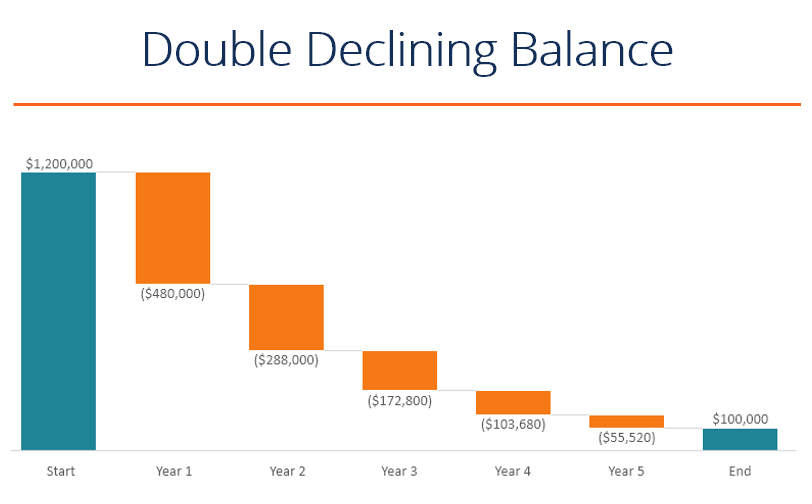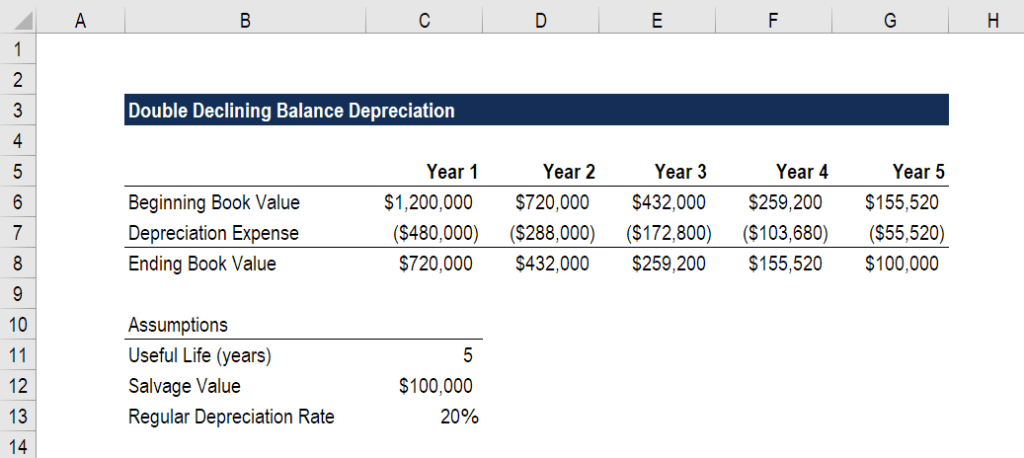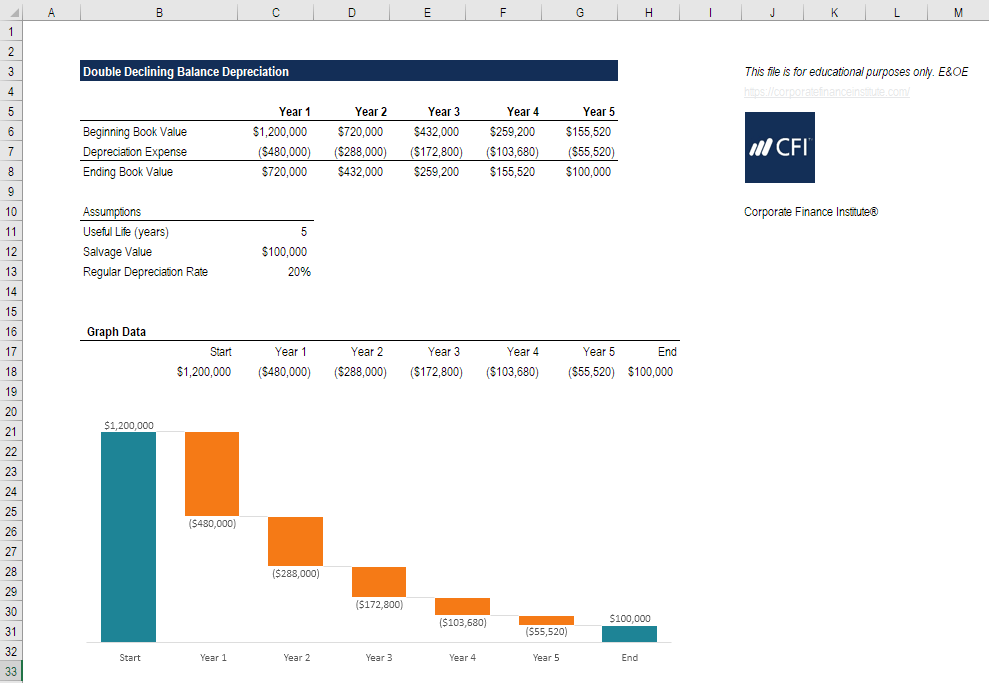# Double Declining Balance Depreciation

An accelerated depreciation method that uses a multiple twice that of the regular declining balance method

## What is the Double Declining Balance Depreciation Method?

The double declining balance depreciation method is a form of accelerated depreciation that doubles the regular depreciation approach. It is frequently used to depreciate fixed assets more heavily in the early years, which allows the company to defer income taxes to later years. This guide will explain how it works and provide examples.### How to calculate the double declining balance

Let’s examine the steps that need to be taken to calculate this form of accelerated depreciation.

#### Steps:

1. Obtain the beginning book value of the asset (e.g., \$1,200,000).
2. Determine the useful life of the asset (e.g., 5 years).
3. Determine the salvage value of the asset (e.g., \$200,000).
4. Deduct the salvage value from the from the beginning book value to determine the total depreciable amount for the life of the asset (i.e., \$1,200,000 – \$100,000 = \$1,100,000).
5. Calculate the annual depreciation rate (i.e., 100% / 5 years = 20%).
6. Multiply the beginning period book value by twice the regular annual rate (\$1,200,000 x 40% = \$480,000).
7. Deduct the annual depreciation expense from the beginning period value to calculate the ending period value.
8. Repeat the above steps until the salvage value is reached.

### Example of double declining balance depreciation in Excel

Using the steps outlined above, let’s walk through an example of how to build a table that calculates the full depreciation schedule over the life of the asset.

Look at the screenshot below and then read the explanation of how it works.As you can see in the Excel analysis, a few key assumptions have to be made, and from there, an analyst can build the full schedule.

Key assumptions include:

• Beginning book value
• Useful life
• Salvage value

Next, an analyst builds the depreciation schedule with the following steps:

• Enter the beginning value.
• Calculate depreciation expense:
• Logic: Beginning value x depreciation rate x 2
• Formula: =MAX(-C6*\$C\$13*2,-(C6-\$C\$12))
• The extra piece that’s added here is that in year 5, only the difference between the opening value and salvage value should be expensed. If more than that were expensed, the closing value would go below the salvage value.
• Calculate the closing value.

Download the free Excel double declining balance template to play with the numbers and calculate double declining balance depreciation expense on your own! The best way to understand how it works is to use your own numbers and try building the schedule yourself.### How the tax advantage works

By accelerating the depreciation method and incurring a larger expense in earlier years and a smaller expense in later years, taxes are pushed out. Let’s look at an example below of how this works.

Continuing with the same numbers as the example above, in year 1 the company would have depreciation of \$480,000 under the accelerated approach, but only \$240,000 under the normal declining balance approach.

In year 5, however, the balance would shift and the accelerated approach would have only \$55,520 of depreciation, while the non-accelerated approach would have a higher number.

The total expense over the life of the asset will be the same under both approaches. The only difference is the timing of the expense.

Since depreciation is tax-deductible (and, therefore, lowers a company’s net income), the company pays less in taxes in the early years and higher taxes in the later years with the double declining balance method.

### Alternative methods

There are various alternative methods that can be used for calculating a company’s annual depreciation expense.

Common methods include:

• Straight-line
• Declining balance
• Double declining balance
• Units of production
• Sum of years digits

Essentially, companies can use whatever method they please, provided they offer some explanation for why they use that method.

Companies will typically keep two sets of books (two sets of financial statements) – one for tax filings, and one for investors. Companies can (and do) use different depreciation methods for each set of books. For tax purposes, they want the expense to be high (to lower taxes). For investors, they want deprecation to be low (to show higher profits).

Thank you for reading this guide to the purpose behind the double declining balance depreciation method.

CFI is the official global provider of the Financial Modeling & Valuation Analyst (FMVA)TMdesigned to transform anyone into a world-class financial analyst.  To continue advancing your career, these additional CFI resources will be helpful:

• How to become a financial analyst
• Analysis of financial statements
• Financial modeling guide
• All accounting resources